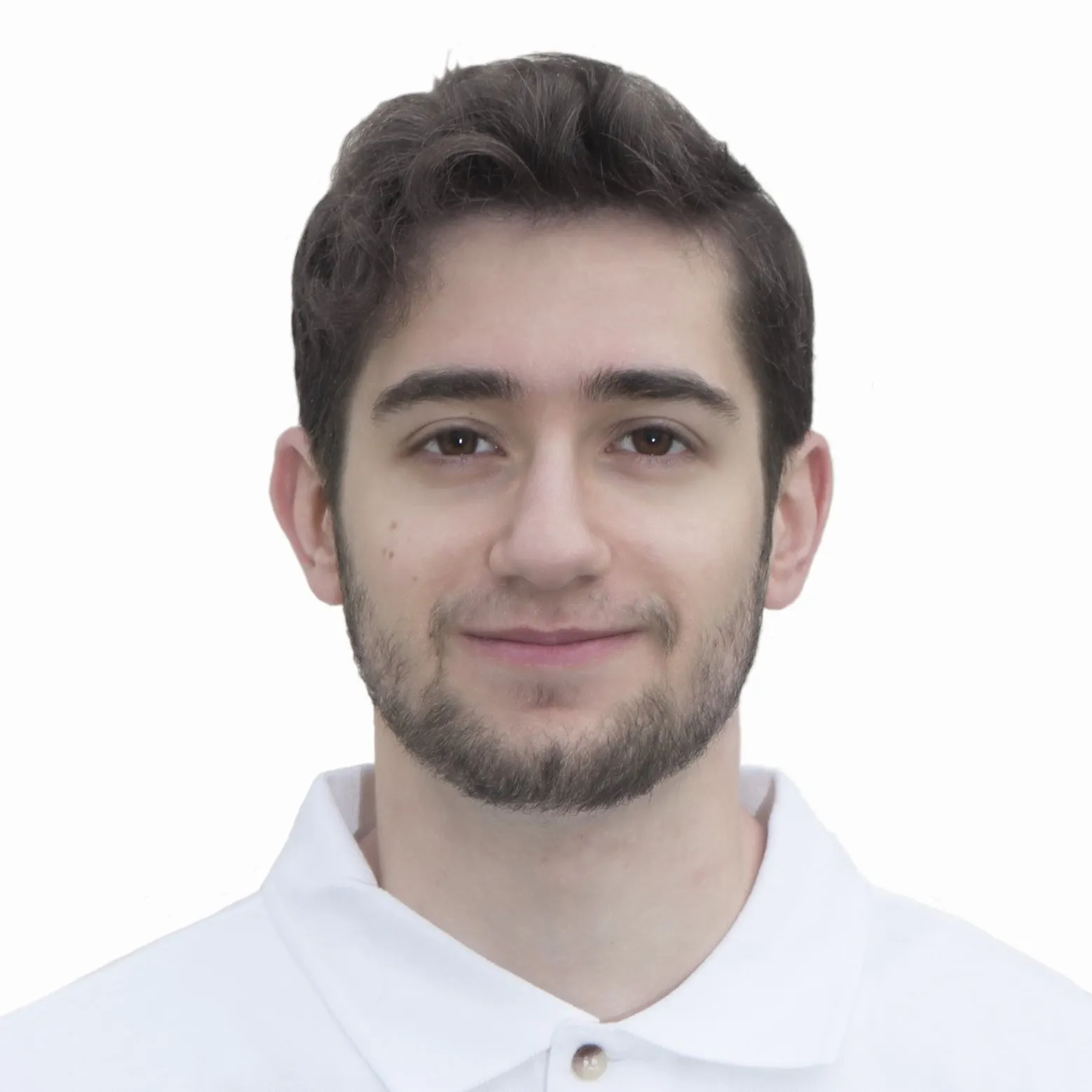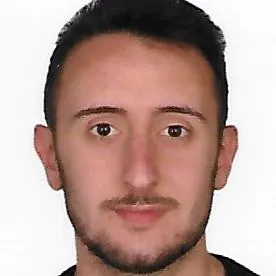A system that aims to satisfy today’s urgent need for mass behavioral monitoring, highly beneficial in the Covid-19 pandemic scenario.

ExpertFull instructions provided3 hours1,246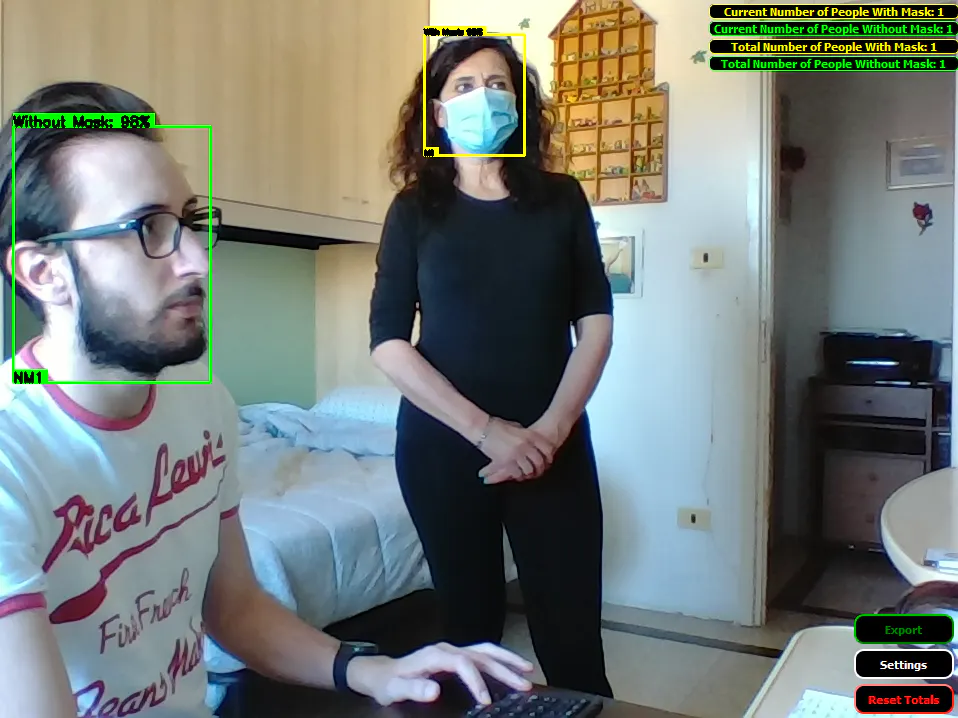## Things used in this project

### Software apps and online services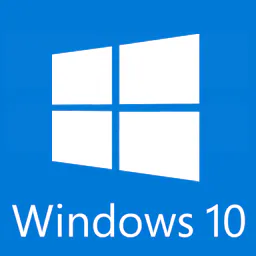Microsoft Windows 10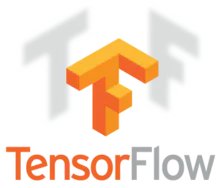TensorFlow
 NVIDIA CUDA Toolkit v10.0
 NVIDIA CuDNN v7.6.5
 Python 3

## Code

### Desktop App

Python
# Import packages
import os
import cv2
import numpy as np
import tensorflow as tf
import sys
import time
import json
from copy import deepcopy
from datetime import datetime,date, timedelta
import pickle

from PyQt5.QtWidgets import *
from PyQt5.QtGui import *
from PyQt5.QtCore import *

from playsound import playsound

from lib.tracker import Tracker
from lib.reports import *
from lib.proximity_detector import *

class ObjectDetection(QMainWindow):

def __init__(self, *args, **kwargs):
super(ObjectDetection, self).__init__(*args, **kwargs)

self.DATA_FILE='data/DATA.bin'
self.CONFIG_FILE='data/CONFIG.json'

self.running=True
self.opened_settings=False

self.crowd_list={}
self.alarm_time=1 # 1 second
self.alarm_list=set()
self.alarm_on=False

self.t1=time.time()
self.t2=time.time()
self.t3=time.time()

if os.path.exists(self.DATA_FILE):
f = open(self.DATA_FILE, "rb")

if True:
if self.DATA!={}:
last=datetime.datetime.utcfromtimestamp(list(self.DATA.keys())[-1])
now=datetime.datetime.now()
tmp=[now.year,now.month,now.day,now.hour]
tmp2=[last.year,last.month,last.day,last.hour]
new=last
while tmp2!=tmp:
new= new + datetime.timedelta(seconds = 3600)
tmp2=[new.year,new.month,new.day,new.hour]
timestamp=new.timestamp()
timestamp=round(timestamp,6)
self.DATA[timestamp]={"NM":[],"M":[]}
else:
self.DATA={}
f.close()
else:
self.DATA={}

with open(self.CONFIG_FILE) as json_file:

# Import settings from configuration file
self.maxAbsences=self.CONFIGS["maxAbsences"]
self.categories =self.CONFIGS["categories"]
self.colors =[tuple(x) for x in self.CONFIGS["colors"]]
self.min_scores=self.CONFIGS["min_score"]
self.border_pxl=self.CONFIGS["border"]
self.models_path=self.CONFIGS["models_path"]
self.models=os.listdir(self.models_path)
self.models=['.'.join(x.split('.')[:-1]) for x in self.models]
self.default_model=self.CONFIGS["default_model"]
self.min_score=self.min_scores[self.default_model]
self.path_to_ckpt = self.models_path+'\\'+self.default_model+'.pb'
self.show_scores=bool(self.CONFIGS["show_scores"])
self.show_IDs=bool(self.CONFIGS["show_IDs"])

# Load counts from temp directory
if os.path.exists(self.tmppath):
with open(self.tmppath, 'r') as file:
self.cntMTot = int(data)
self.cntVTot = int(data)
else:
with open(self.tmppath, 'w+') as file:
self.cntMTot=0
self.cntVTot=0
file.write('%d,%d' %(self.cntMTot, self.cntVTot))

# Init an istance of a tracker for each class

self.font = cv2.FONT_HERSHEY_SIMPLEX
self.setWindowTitle('COVIDEO v3')
self.setWindowIcon(QIcon('img\\Icon.jpg'))
self.setGeometry(100, 100, 1280, 720)
self.setStyleSheet("background-color:white")
self.setMinimumWidth(1280)
self.setMaximumWidth(1280)
self.setMaximumHeight(720)
self.setMinimumHeight(720)
self.pic = QLabel(self)
self.pic.setGeometry(0, 0, 1280, 720)
self.logo = QLabel(self)
self.logo.setGeometry(0, 690, 180, 30)
self.logo.setStyleSheet("background-color:transparent;")
self.logo.setPixmap(QPixmap('img\\logo_white.png'))
self.logo.setScaledContents(True)
self.textCM = QPushButton(self)

self.textCM.setStyleSheet("background-color:black; color:"+('#%02x%02x%02x' % self.colors)+"; font:bold; border-style:outset;\
self.textCM.setText('Current Number of People '+self.categories+': 0')
self.textCM.setGeometry(1030, 5, 250, 15)
self.textCV = QPushButton(self)

self.textCV.setStyleSheet("background-color:black; color:"+('#%02x%02x%02x' % self.colors)+"; font:bold; border-style:outset;\
self.textCV.setText('Current Number of People '+self.categories+': 0')
self.textCV.setGeometry(1030, 22.5, 250, 15)
self.textTM = QPushButton(self)

self.textTM.setStyleSheet("background-color:black; color:"+('#%02x%02x%02x' % self.colors)+"; font:bold; border-style:outset;\
self.textTM.setText('Total Number of People '+self.categories+': 0')
self.textTM.setGeometry(1030, 40, 250, 15)
self.textTV = QPushButton(self)

self.textTV.setStyleSheet("background-color:black; color:"+('#%02x%02x%02x' % self.colors)+"; font:bold; border-style:outset;\
self.textTV.setText('Total Number of People '+self.categories+': 0')
self.textTV.setGeometry(1030, 57.5, 250, 15)
self.b1 = QPushButton(self)

self.b1.setStyleSheet("QPushButton{background-color:black; color:red; font:bold; border-style:outset;\
"QPushButton:hover{background-color:red; color:black; font:bold; border-style:outset;\
"QPushButton:pressed{background-color:black; color:red; font:bold; border-style:outset;\

self.b1.clicked.connect(self.reset_cnts)
self.b1.setText('Reset Totals')
self.b1.setGeometry(1175, 685, 100, 30)

self.b2 = QPushButton(self)
self.b2.setStyleSheet("QPushButton{background-color:black; color:white; font:bold; border-style:outset;\
"QPushButton:hover{background-color:white; color:black; font:bold; border-style:outset;\
"QPushButton:pressed{background-color:black; color:white; font:bold; border-style:outset;\
self.b2.clicked.connect(self.change_settings)
self.b2.setText('Settings')
self.b2.setGeometry(1175, 650, 100, 30)

self.b3 = QPushButton(self)
self.b3.setStyleSheet("QPushButton{background-color:black; color:green; font:bold; border-style:outset;\
"QPushButton:hover{background-color:green; color:black; font:bold; border-style:outset;\
"QPushButton:pressed{background-color:black; color:green; font:bold; border-style:outset;\
self.b3.clicked.connect(self.export)
self.b3.setText('Export')
self.b3.setGeometry(1175, 615, 100, 30)

self.category_index={1: {'id': 1, 'name': self.categories}, 2: {'id': 2, 'name': self.categories}}

# Run main functions
self.define_io_tensors()
self.video_setting()
self.show()
self.start()

def closeEvent(self, event):
# close app
close = QMessageBox.question(self,"QUIT","Are you sure you want to stop the process?",QMessageBox.Yes | QMessageBox.No)
if close == QMessageBox.Yes:
self.save_data()
self.video.release()
cv2.destroyAllWindows()
event.accept()
else:
event.ignore()

def reset_cnts(self):
# Reset data
self.running=False
ans = QMessageBox.question(self,"RESET","Are you sure you want to reset the total counts?",QMessageBox.Yes | QMessageBox.No)
if ans == QMessageBox.Yes:
self.cntMTot=0
self.cntVTot=0
f=open(self.DATA_FILE, 'wb')
f.close()
self.DATA={}
else:
pass
self.running=True
self.start()

def selectionchange(self):
selected=self.comboBox.currentText()
self.minscoreEdit.setText(str(self.min_scores[selected]*100))

def change_settings(self):

# Graphical interface for settings

self.win = QWidget()
self.win.setWindowIcon(QIcon('img\\Icon.jpg'))
self.maxabsLabel = QLabel("Maximum number of absences:")
self.maxabsEdit = QLineEdit()
self.maxabsEdit.setText(str(self.maxAbsences))
self.minscoreLabel = QLabel("Minimum score (%):")
self.minscoreEdit = QLineEdit()
self.minscoreEdit.setText(str(self.min_score*100))
self.borderLabel = QLabel("Pixels of border:")
self.borderEdit = QLineEdit()
self.borderEdit.setText(str(self.border_pxl))
self.modelLabel = QLabel("Model:")
self.comboBox = QComboBox()
self.comboBox.setCurrentIndex(self.models.index(self.default_model))
self.comboBox.currentIndexChanged.connect(self.selectionchange)

self.colorLabe0 = QLabel(self.categories+" color:")
self.color0_button = QPushButton()
self.color0_button.clicked.connect(lambda: self.get_color(0))
self.color0_button.setStyleSheet("background-color:rgb"+str(self.colors))

self.colorLabel1 = QLabel(self.categories+" color:")
self.color1_button = QPushButton()
self.color1_button.clicked.connect(lambda: self.get_color(1))
self.color1_button.setStyleSheet("background-color:rgb"+str(self.colors))

self.checkbox1 = QCheckBox("Show scores")
self.checkbox1.setChecked(self.show_scores)
self.checkbox2 = QCheckBox("Show IDs")
self.checkbox2.setChecked(self.show_IDs)

self.ok = QPushButton()
self.ok.setText('Save')
self.ok.clicked.connect(self.restart)

self.Label1 = QLabel("- OBJECT DETECTION:")
self.Label1.setStyleSheet("font-weight: bold")
self.Label2 = QLabel("- TRACKING:")
self.Label2.setStyleSheet("font-weight: bold")
self.Label3 = QLabel("- DESIGN:")
self.Label3.setStyleSheet("font-weight: bold")
self.Label4 = QLabel("")

# Put the widgets in a layout (now they start to appear):
self.layout = QGridLayout()

self.win.setLayout(self.layout)
self.win.setGeometry(100,100,300,300)
self.win.setWindowTitle("Settings")
self.tmp_colors=[deepcopy(x) for x in self.colors]
self.win.show()

def get_color(self,i):
self.tmp_colors[i] = QColorDialog.getColor().getRgb()
if i ==0:
self.color0_button.setStyleSheet("background-color:rgb"+str(self.tmp_colors[i]))

else:
self.color1_button.setStyleSheet("background-color:rgb"+str(self.tmp_colors[i]))

def restart(self):
self.running=False
try:
tmp=int(self.maxabsEdit.text())
if tmp>=0:
self.maxAbsences=tmp

except:
self.maxabsEdit.setText(str(self.maxAbsences))

try:
tmp=float(self.minscoreEdit.text())
if tmp>=0 and tmp<=100:
self.min_score=tmp/100
except:
self.minscoreEdit.setText(str(self.min_score*100))

try:
tmp=int(self.borderEdit.text())
if tmp>=0:
self.border_pxl=tmp
except:
self.maxabsEdit.setText(str(self.border_pxl))

self.border_pxl=int(self.borderEdit.text())
self.colors=[tuple(list(x)[:3]) for x in self.tmp_colors]

self.textCM.setStyleSheet("background-color:black; color:"+('#%02x%02x%02x' % self.colors)+"; font:bold; border-style:outset;\
self.textCV.setStyleSheet("background-color:black; color:"+('#%02x%02x%02x' % self.colors)+"; font:bold; border-style:outset;\
self.textTM.setStyleSheet("background-color:black; color:"+('#%02x%02x%02x' % self.colors)+"; font:bold; border-style:outset;\
self.textTV.setStyleSheet("background-color:black; color:"+('#%02x%02x%02x' % self.colors)+"; font:bold; border-style:outset;\

if self.checkbox1.isChecked():
self.show_scores=True
else:
self.show_scores=False

if self.checkbox2.isChecked():
self.show_IDs=True
else:
self.show_IDs=False

if self.default_model!=self.comboBox.currentText():
self.default_model=self.comboBox.currentText()
self.path_to_ckpt = self.models_path+'\\'+self.default_model+'.pb'
self.define_io_tensors()

self.running=True
self.start()

def is_valid_filename(self,filename):
invalid=['\\', '/', ':', '*', '?', '"', '<', '>', '|']
for i in invalid:
if i in filename:
return False
return True

def export(self):
# Generate and export statistics
date=datetime.datetime.now()
name = QFileDialog.getSaveFileName(self, 'Save File', str(date.strftime("%Y-%m-%d_%H-%M-%S")), "XLSX (*.xlsx)")
if name=='' or self.DATA=={}:
pass

elif self.is_valid_filename(name.split('/')[-1]):
export_records(name, self.DATA)
else:
self.export()

# Load the Tensorflow model into memory.
self.detection_graph = tf.Graph()
with self.detection_graph.as_default():
od_graph_def = tf.compat.v1.GraphDef()
with tf.io.gfile.GFile(self.path_to_ckpt, 'rb') as fid:
od_graph_def.ParseFromString(serialized_graph)
tf.import_graph_def(od_graph_def, name='')

self.sess = tf.compat.v1.Session(graph=self.detection_graph)

def define_io_tensors(self):
# Define input and output tensors (i.e. data) for the object detection classifier
# Input tensor is the image
self.image_tensor = self.detection_graph.get_tensor_by_name('image_tensor:0')
# Output tensors are the detection boxes, scores, and classes
# Each box represents a part of the image where a particular object was detected
self.detection_boxes = self.detection_graph.get_tensor_by_name('detection_boxes:0')
# Each score represents level of confidence for each of the objects.
# The score is shown on the result image, together with the class label.
self.detection_scores = self.detection_graph.get_tensor_by_name('detection_scores:0')
self.detection_classes = self.detection_graph.get_tensor_by_name('detection_classes:0')
# Number of objects detected
self.num_detections = self.detection_graph.get_tensor_by_name('num_detections:0')

def video_setting(self):
# Initialize webcam feed
self.video = cv2.VideoCapture(0)
self.ret = self.video.set(3, 1280)
self.ret = self.video.set(4, 720)

def save_data(self):
# Save data
f=open(self.DATA_FILE, 'wb')
pickle.dump(self.DATA,f)
f.close()

with open(self.tmppath, 'w+') as f:
f.write('%d,%d' %(self.cntMTot, self.cntVTot))

def start(self):
time.sleep(2)

while(self.running):

try:
# Acquire frame and expand frame dimensions to have shape: [1, None, None, 3]
# i.e. a single-column array, where each item in the column has the pixel RGB value
frame_rgb = cv2.cvtColor(frame, cv2.COLOR_BGR2RGB)
frame_expanded = np.expand_dims(frame_rgb, axis=0)

# Perform the actual detection by running the model with the image as input
(boxes, scores, classes, num) = self.sess.run(
[self.detection_boxes, self.detection_scores, self.detection_classes, self.num_detections],
feed_dict={self.image_tensor: frame_expanded})

faces=[]
labels=[]
for i,score in enumerate(scores):
if score>self.min_score:
ymin=int(boxes[i]*frame.shape)
xmin=int(boxes[i]*frame.shape)
ymax=int(boxes[i]*frame.shape)
xmax=int(boxes[i]*frame.shape)
lab=self.categories[int(classes[i])-1]
faces.append([xmin,xmax,ymin,ymax])
labels.append(lab)

ind=int(classes[i])-1
# Draw the results of the detection
cv2.rectangle(frame, (xmin, ymin), (xmax, ymax),tuple((list(self.colors[ind])[:3])[::-1]), 2)
if self.show_scores:
text=lab+': '+str(int(score*100))+'%'
font_scale = (xmax-xmin)/frame.shape*3
(text_width, text_height) = cv2.getTextSize(text, self.font, fontScale=font_scale, thickness=2)
text_offset_x = xmin
text_offset_y = ymin
box_coords = ((text_offset_x, text_offset_y), (text_offset_x + text_width + 2, text_offset_y - text_height - 2))
cv2.rectangle(frame, box_coords, box_coords, tuple((list(self.colors[ind])[:3])[::-1]), cv2.FILLED)
cv2.putText(frame, text, (text_offset_x, text_offset_y), self.font, fontScale=font_scale, color=(0, 0, 0), thickness=2,lineType=2)

# Get the list of all masked faces
masked_faces=[faces[i] for i, x in enumerate(labels) if x == self.categories]
# Get the list of all not masked faces
unmasked_faces=[faces[i] for i, x in enumerate(labels) if x == self.categories]
# Tracking

# Check proximity between people

# if near people are detected, results are reported and sound and graphical alarms are activated
for r in result:
if r in list(self.crowd_list.keys()):
if (time.time()-self.crowd_list[r])>self.alarm_time:

else:
self.crowd_list[r]=time.time()
tmp=deepcopy(self.crowd_list)
for k in list(tmp.keys()):
if k not in result:
del self.crowd_list[k]
if k in self.alarm_list:
self.alarm_list.remove(k)

# run sound alarm
if len(self.alarm_list)>0 and not self.alarm_on:

# loop over the tracked masked people
# draw both the ID of the object and the centroid of the
# object on the output frame
if self.show_IDs:
xmin,xmax,ymin,ymax=box
text = "M{}".format(objectID)
font_scale = (xmax-xmin)/frame.shape*3
(text_width, text_height) = cv2.getTextSize(text, self.font, fontScale=font_scale, thickness=2)
text_offset_x = xmin
text_offset_y = ymax
box_coords = ((text_offset_x, text_offset_y), (text_offset_x + text_width + 2, text_offset_y - text_height - 2))
cv2.rectangle(frame, box_coords, box_coords, tuple((list(self.colors)[:3])[::-1]), cv2.FILLED)
cv2.putText(frame, text, (text_offset_x, text_offset_y), self.font, fontScale=font_scale, color=(0, 0, 0), thickness=2,lineType=2)
# if people are too near, a red rectangle is drawn
for alarm in self.alarm_list:
if alarm==text or alarm==text:
cv2.rectangle(frame, (xmin,ymin), (xmax,ymax), (0,0,255), thickness = 5)
if objectID>self.cntMTot:
self.cntMTot=objectID

# loop over the tracked unmasked people
# draw both the ID of the object and the centroid of the
# object on the output frame
if self.show_IDs:
xmin,xmax,ymin,ymax=box
text = "NM{}".format(objectID)
font_scale = (xmax-xmin)/frame.shape*3
(text_width, text_height) = cv2.getTextSize(text, self.font, fontScale=font_scale, thickness=2)
text_offset_x = xmin
text_offset_y = ymax
box_coords = ((text_offset_x, text_offset_y), (text_offset_x + text_width + 2, text_offset_y - text_height - 2))
cv2.rectangle(frame, box_coords, box_coords, tuple((list(self.colors)[:3])[::-1]), cv2.FILLED)
cv2.putText(frame, text, (text_offset_x, text_offset_y), self.font, fontScale=font_scale, color=(0, 0, 0), thickness=2,lineType=2)
# if people are too near, a red rectangle is drawn
for alarm in self.alarm_list:
if alarm==text or alarm==text:
cv2.rectangle(frame, (xmin,ymin), (xmax,ymax), (0,0,255), thickness = 5)
# update total number of unmasked
if objectID>self.cntVTot:
self.cntVTot=objectID

# Update graphical statistics
self.textCM.setText('Current Number of People '+self.categories+': %d' %self.cntM)
self.textCV.setText('Current Number of People '+self.categories+': %d' %self.cntV)
self.textTM.setText('Total Number of People '+self.categories+': %d' %self.cntMTot)
self.textTV.setText('Total Number of People '+self.categories+': %d' %self.cntVTot)

self.timestamp=time.time()
# Save samples each second
if self.timestamp-self.t1>1:
self.t1=self.timestamp

# Write data to file every 60 seconds
if self.timestamp-self.t2>60:
self.save_data()
self.t2=self.timestamp

if not self.isVisible():
exit()

# All the results have been drawn on the frame, so it's time to display it.
height, width, channel = frame.shape
bytesPerLine = 3 * width
self.qImg = QImage(frame.data, width, height, bytesPerLine, QImage.Format_RGB888).rgbSwapped()
self.pic.setPixmap(QPixmap(self.qImg))
if cv2.waitKey(1) == ord('q'):
break

except:
with open(self.tmppath, 'w+') as file:
file.write('%d,%d' %(self.cntMTot, self.cntVTot))
exit()

def alarm(self):
self.alarm_on=True
playsound('data/beep.mp3')
self.alarm_on=False

if __name__=='__main__':

app = QApplication(sys.argv)

window = ObjectDetection()
window.show()

app.exec_()


### Tracker

Python
import numpy as np
from scipy.spatial import distance as dist
from copy import deepcopy

class Tracker():
def __init__(self, maxAbsences=50, startID=1):

# ID Counter
self.nextID = startID

# To store current objects
self.objects = {}

# To count how many consecutive frames an object is absent
self.absences = {}

# To store coordinates to return
self.coordinates={}

# To backup
self.coordinates_backup={}

# Maximum number of consecutive absences of an object before its deletion
self.maxAbsences = maxAbsences

# Add a new detected object and increases object counter
self.objects[self.nextID] = centroid
self.absences[self.nextID] = 0
self.nextID += 1

def remove(self, objectID):
# Remove a disappeared object
del self.objects[objectID]
del self.absences[objectID]

def refresh(self, inputObjects, imgSize, border):

# If the list of input objects of the current frame is empty:
if len(inputObjects) == 0:
# It is necessary to add an absense to every object present in the archive
for objectID in list(self.absences.keys()):
self.absences[objectID] += 1

# If an object is absent and in the last presence was at the boundaries of the picture
# it will be deleted
x_min, x_max, y_min, y_max = self.coordinates_backup[objectID]
if ((x_min in range(-50,border))
or (x_max in range(imgSize-border,imgSize+50))
or (y_min in range(-50,border))
or (y_max in range(imgSize-border,imgSize+50))):
self.remove(objectID)
del self.coordinates_backup[objectID]

# If an object has reached the maximum number of consecutive absences, it is deleted
elif self.absences[objectID] > self.maxAbsences:
self.remove(objectID)

self.coordinates={}

return self.coordinates

# Compute centroids for the objects in the current frame
inputCentroids = [self.calc_centroid(inputObject) for inputObject in inputObjects]

# if there are no objects in the archive, all the input objects are added
if len(self.objects) == 0:
for i in range(0, len(inputCentroids)):
self.coordinates[self.nextID]=inputObjects[i]
self.coordinates_backup[self.nextID]=inputObjects[i]

# else it necessary to match the input objects with the already existing ones
else:
objectIDs = list(self.objects.keys())
objectCentroids = list(self.objects.values())

# compute distances between input objects and the already existing ones
D = dist.cdist(np.array(objectCentroids), np.asarray(inputCentroids))

# Sort and match by distance
rows = D.min(axis=1).argsort()
cols = D.argmin(axis=1)[rows]

usedRows = set()
usedCols = set()

for (row, col) in zip(rows, cols):
if row in usedRows or col in usedCols:
continue
#else take for the current row the object ID and set its
# updated centroid and reset its absences
objectID = objectIDs[row]
self.objects[objectID] = inputCentroids[col]
self.coordinates[objectID]=inputObjects[col]
self.coordinates_backup[objectID]=inputObjects[col]
self.absences[objectID] = 0

# get the unused rows indexes (correspondent to objects that need
# to be removed)
unusedRows = set(range(0, D.shape)).difference(usedRows)
#get the unused columns indexes (correspondent to the input objects
unusedCols = set(range(0, D.shape)).difference(usedCols)

# if the number of input objects is equal or lower than the number of
# objects in the archive, it means that some objects are absent
if D.shape >= D.shape:
for row in unusedRows:
objectID = objectIDs[row]
self.absences[objectID] += 1
x_min, x_max, y_min, y_max = self.coordinates_backup[objectID]

# if in the preview frame an absent objet was at the
#boundary of the image, it needs to be deleted from the archive
# (object exiting from the picture)
if ((x_min in range(-border*4,border))
or (x_max in range(imgSize-border,imgSize+border*4))
or (y_min in range(-border*4,border))
or (y_max in range(imgSize-border,imgSize+border*4))):
self.remove(objectID)
del self.coordinates_backup[objectID]
del self.coordinates[objectID]
# an object has reached the maximum number of consecutive absenses
# needs to be deleted
elif self.absences[objectID] > self.maxAbsences:
self.remove(objectID)
if objectID in list(self.coordinates.keys()):
del self.coordinates[objectID]
# else if the number of input objects is greater than the number of
#objects in the archive of objects, new objects need to be added
else:
for col in unusedCols:
self.coordinates[self.nextID]=inputObjects[col]
self.coordinates_backup[self.nextID]=inputObjects[col]

return self.coordinates

def calc_centroid(self,detection):
# calculate the centroid
x_min, x_max, y_min, y_max = detection
return [int((x_min+x_max)/2.0),int((y_min+y_max)/2.0)]

def reset(self):
self.nextID = 1
self.objects = {}
self.absences = {}
self.coordinates={}


### Proximity Detector

Python
import math

# function that receives the model output.
# having the ID of each face (masked or bare) as
# keys, the coordinates of the boxes containing the
# faces as values (i.e. ID 3 => [0, 200, 0, 200]).
# coordinates are expressed as [xmin, xmax, ymin, ymax].
result = set()
std_ratio = 2 / 3 # standard parameter
std_face_width = 0.16 # standard face width in meters

# is there anyone that does not have a mask? If yes, verify that no one is close to him!
first_width = item - item
first_height = item - item
first_max = max(first_width, first_height)
first = (item + first_width / 2, item + first_height / 2) # x,y coordinates of NM's centroid

# are there any masked people close to the one who's not masked?
second_width = m - m
second_height = m - m
second_max = max(second_width, second_height)
second = (m + second_width / 2, m + second_height / 2) # x,y coordinates of M's centroid
if (first_max <= second_max and first_max > second_max * std_ratio) or\
(second_max < first_max and second_max > first_max * std_ratio): # are the faces' depths comparable?
distance = math.sqrt((first - second)**2 + (first - second)**2)
if distance / ((first_max + second_max) / 2) < 1 / std_face_width: # people are too close to each other!
result.add(tuple(sorted(('NM{}'.format(item), 'M{}'.format(m))))) # tuple+sorted combo is for preventing simmetries

# are there any unmasked people close to the one who's not masked?
if nm != item:
second_width = nm - nm
second_height = nm - nm
second_max = max(second_width, second_height)
second = (nm + second_width / 2, nm + second_height / 2) # x,y coordinates of M's centroid
if (first_max <= second_max and first_max > second_max * std_ratio) or\
(second_max < first_max and second_max > first_max * std_ratio): # are the faces' depths comparable?
distance = math.sqrt((first - second)**2 + (first - second)**2)
if distance / ((first_max + second_max) / 2) < 1 / std_face_width: # people are too close to each other!
result.add(tuple(sorted(('NM{}'.format(item), 'NM{}'.format(nm))))) # tuple+sorted combo is for preventing simmetries

return result


### Reports

Python
import xlsxwriter
import json
import datetime
import itertools
from random import *
import time
import pickle
import calendar

f = open(filename, "rb")
f.close()
return data

def timestamps_to_datetimes(data):
# Convert timestamps in datetimes objects
timestamps=list(data.keys())
datetime_objects = [datetime.datetime.fromtimestamp(timestamp) for timestamp in timestamps]
return datetime_objects

def split_datetimes_by_year(datetime_objects):
# Separate datetimes objects by year
return [list(g) for k, g in itertools.groupby(datetime_objects, key=lambda d: d.year)]

def split_datetimes_by_month(datetime_objects):
# Separate datetimes objects by month
return [list(g) for k, g in itertools.groupby(datetime_objects, key=lambda d: d.month)]

def split_datetimes_by_day(datetime_objects):
# Separate datetimes objects by day
return [list(g) for k, g in itertools.groupby(datetime_objects, key=lambda d: d.day)]

def split_datetimes_by_hour(datetime_objects):
# Separate datetimes objects by hour
return [list(g) for k, g in itertools.groupby(datetime_objects, key=lambda d: d.hour)]

def prepare_for_monthly_chart(data, key=["M","NM"]):
# Split data by month
months_names=['Jan', 'Feb', 'Mar', 'Apr', 'May', 'Jun', 'Jul', 'Aug', 'Sep', 'Oct', 'Nov', 'Dec']
datetime_objects = timestamps_to_datetimes(data)
dt_by_month = split_datetimes_by_month(datetime_objects)
months=[]
for month in dt_by_month:

months.append(datetime.datetime(month.year,month.month,1))
m_samples=[]
nm_samples=[]
for sample in month:

timestamp = sample.timestamp()
m_samples=m_samples+data[timestamp][key]
nm_samples=nm_samples+data[timestamp][key]

def prepare_for_daily_chart(data, key=["M","NM"]):
# Split data by day
datetime_objects = timestamps_to_datetimes(data)
dt_by_day = split_datetimes_by_day(datetime_objects)
days=[]
for day in dt_by_day:
days.append(datetime.datetime(day.year,day.month,day.day))
m_samples=[]
nm_samples=[]
for sample in day:
timestamp = sample.timestamp()
m_samples=m_samples+data[timestamp][key]
nm_samples=nm_samples+data[timestamp][key]

def prepare_for_hourly_last_week_chart(data, key=["M","NM"]):
# Take the data of the last week if available and split it by hour
datetime_objects = timestamps_to_datetimes(data)

dt_by_days = split_datetimes_by_day(datetime_objects)

today = dt_by_days[-1]
today_hours=[]

today_by_hour = split_datetimes_by_hour(today)
for h in today_by_hour:
today_hours.append(datetime.datetime(h.year,h.month,h.day,h.hour))
m_samples=[]
nm_samples=[]
for sample in h:
timestamp = sample.timestamp()
m_samples=m_samples+data[timestamp][key]
nm_samples=nm_samples+data[timestamp][key]
if len(dt_by_days)>7:
week_days = dt_by_days[-8:-1]
week_hours=[]
for day in week_days:
week_by_hour = split_datetimes_by_hour(day)
week_hours.append([])
for h in week_by_hour:
week_hours[-1].append(datetime.datetime(h.year,h.month,h.day,h.hour))
m_samples=[]
nm_samples=[]
for sample in h:
timestamp = sample.timestamp()
m_samples=m_samples+data[timestamp][key]
nm_samples=nm_samples+data[timestamp][key]
else:
week_hours=[]

def hourly_last_week_chart(preprocessed_data, workbook,sheetname="hourly_presence",kind='column', dim=[800,600]):
# Function to draw hourly charts on excel

chart=[]
dy=5
dx=0

today_data=preprocessed_data

week_data=preprocessed_data

# get data for the current day
data = today_data

X=[d.strftime("%H") for d in data]

gap=1000

worksheet.write_row('B'+str(dy-1), [data.strftime("%a %d %b %Y")], bold)
worksheet.write_column(chr(ord('A')+dx)+str(gap+1), X)
worksheet.write_column(chr(ord('B')+dx)+str(gap+1), data)
worksheet.write_column(chr(ord('C')+dx)+str(gap+1), data)

# Configure the chart
chart.add_series({'name': '='+sheetname+'!$'+chr(ord('B')+dx)+'$'+str(gap),'categories': '='+sheetname+'!$'+chr(ord('A')+dx)+'$'+str(gap+1)+':$'+chr(ord('A')+dx)+'$'+str(len(data)+2+gap),'values': '='+sheetname+'!$'+chr(ord('B')+dx)+'$'+str(gap+1)+':$'+chr(ord('B')+dx)+'$'+str(len(data)+2+gap)})
chart.add_series({'name': '='+sheetname+'!$'+chr(ord('C')+dx)+'$'+str(gap),'categories': '='+sheetname+'!$'+chr(ord('A')+dx)+'$'+str(gap+1)+':$'+chr(ord('A')+dx)+'$'+str(len(data)+2+gap),'values': '='+sheetname+'!$'+chr(ord('C')+dx)+'$'+str(gap+1)+':$'+chr(ord('C')+dx)+'$'+str(len(data)+2+gap)})

# set chart size
chart.set_size({'width': dim, 'height': dim})

# Insert the chart into the worksheet.
worksheet.insert_chart('B'+str(dy), chart)

dx+=3
dy+=dim/15

n_days=len(week_data)
for i in range(len(week_data)):

# get data for the current day
data=[]
data.append(week_data[n_days-1-i])
data.append(week_data[n_days-1-i])
data.append(week_data[n_days-1-i])

X=[d.strftime("%H") for d in data]

gap=1000

worksheet.write_row('B'+str(dy-1), [data.strftime("%a %d %b %Y")], bold)
worksheet.write_column(chr(ord('A')+dx)+str(gap+1), X)
worksheet.write_column(chr(ord('B')+dx)+str(gap+1), data)
worksheet.write_column(chr(ord('C')+dx)+str(gap+1), data)

# Configure the chart
chart[i+1].add_series({'name': '='+sheetname+'!$'+chr(ord('B')+dx)+'$'+str(gap),'categories': '='+sheetname+'!$'+chr(ord('A')+dx)+'$'+str(gap+1)+':$'+chr(ord('A')+dx)+'$'+str(len(data)+2+gap),'values': '='+sheetname+'!$'+chr(ord('B')+dx)+'$'+str(gap+1)+':$'+chr(ord('B')+dx)+'$'+str(len(data)+2+gap)})
chart[i+1].add_series({'name': '='+sheetname+'!$'+chr(ord('C')+dx)+'$'+str(gap),'categories': '='+sheetname+'!$'+chr(ord('A')+dx)+'$'+str(gap+1)+':$'+chr(ord('A')+dx)+'$'+str(len(data)+2+gap),'values': '='+sheetname+'!$'+chr(ord('C')+dx)+'$'+str(gap+1)+':$'+chr(ord('C')+dx)+'$'+str(len(data)+2+gap)})

# set chart size
chart[i+1].set_size({'width': dim, 'height': dim})

# Insert the chart into the worksheet.
worksheet.insert_chart('B'+str(dy), chart[i+1])

dx+=3
dy+=dim/15

return workbook

def export_records(outputfile, data):
# Function to draw charts on excel

workbook = xlsxwriter.Workbook(outputfile)

monthly_data=prepare_for_monthly_chart(data)
daily_data=prepare_for_daily_chart(data)
hourly_data=prepare_for_hourly_last_week_chart(data)

workbook=hourly_last_week_chart(hourly_data, workbook)

ndays=len(daily_data)
if ndays>7:
workbook=create_chart(workbook, daily_data, "Daily_report","Days",'line')
elif ndays<=7 and ndays>0:
workbook=create_chart(workbook, daily_data, "Daily_report","Days",'column')

nmonths=len(monthly_data)
if nmonths>12:
workbook=create_chart(workbook, monthly_data, "Monthly_report","Months",'line')
elif nmonths<=12 and nmonths>0:
workbook=create_chart(workbook,monthly_data, "Monthly_report","Months",'column')

workbook.close()

def create_chart(workbook, data, sheetname,text,kind,dim=[640,480]):

# Create a new Chart object.

X=[d.strftime("%b %Y") for d in data]

# Add the worksheet data that the charts will refer to.

gap=1000
worksheet.write_row('B2', [sheetname], bold)

worksheet.write_column('A'+str(gap+1), X)
worksheet.write_column('B'+str(gap+1), data)
worksheet.write_column('C'+str(gap+1), data)

# Configure the chart. In simplest case we add one or more data series.
chart.add_series({'name': '='+sheetname+'!$B$'+str(gap),'categories': '='+sheetname+'!$A$'+str(gap+1)+':$A$'+str(len(data)+2+gap),'values': '='+sheetname+'!$B$'+str(gap+1)+':$B$'+str(len(data)+2+gap)})
chart.add_series({'name': '='+sheetname+'!$C$'+str(gap),'categories': '='+sheetname+'!$A$'+str(gap+1)+':$A$'+str(len(data)+2+gap),'values': '='+sheetname+'!$C$'+str(gap+1)+':$C$'+str(len(data)+2+gap)})

chart.set_size({'width': dim, 'height': dim})

# Insert the chart into the worksheet.
worksheet.insert_chart('B4', chart)

# Create a new Chart object.

# Add the worksheet data that the charts will refer to.

worksheet.write_row('B40', ["Percentage of people with mask"], bold)

perc=[data[i]/(data[i]+data[i])*100 if (data[i]!=0 or data[i]!=0) else 0 for i in range(len(data))]
worksheet.write_column('D'+str(gap+1), X)
worksheet.write_column('E'+str(gap+1), perc)

# Configure the chart. In simplest case we add one or more data series.
chart2.add_series({'name': '='+sheetname+'!$E$'+str(gap),'categories': '='+sheetname+'!$D$'+str(gap+1)+':$D$'+str(len(data)+2+gap),'values': '='+sheetname+'!$E$'+str(gap+1)+':$E$'+str(len(data)+2+gap)})

chart2.set_size({'width': dim, 'height': dim})

# Insert the chart into the worksheet.
worksheet.insert_chart('B42', chart2)

return workbook


### FaceDetectorXML.py

Python
import os
from matplotlib import pyplot
import progressbar
import sys
import numpy as np
import tkinter as tk
import tensorflow as tf
from object_detection.utils import label_map_util

# Settings
MIN_SCORE=0.85
PATH_TO_CKPT="../../RCNN/frozen_inference_graph_v3.pb"
PATH_TO_LABEL="../../RCNN/label_map.pbtxt"

categories = label_map_util.convert_label_map_to_categories(label_map, max_num_classes=n_classes, use_display_name=True)
category_index = label_map_util.create_category_index(categories)

# Load the Tensorflow model into memory.
detection_graph = tf.Graph()
with detection_graph.as_default():
od_graph_def = tf.compat.v1.GraphDef()
with tf.io.gfile.GFile(path_to_ckpt, 'rb') as fid:
od_graph_def.ParseFromString(serialized_graph)
tf.import_graph_def(od_graph_def, name='')

sess = tf.compat.v1.Session(graph=detection_graph)

# Define input and output tensors (i.e. data) for the object detection classifier

# Input tensor is the image
image_tensor = detection_graph.get_tensor_by_name('image_tensor:0')

# Output tensors are the detection boxes, scores, and classes
# Each box represents a part of the image where a particular object was detected
detection_boxes = detection_graph.get_tensor_by_name('detection_boxes:0')

# Each score represents level of confidence for each of the objects.
# The score is shown on the result image, together with the class label.
detection_scores = detection_graph.get_tensor_by_name('detection_scores:0')
detection_classes = detection_graph.get_tensor_by_name('detection_classes:0')

# Number of objects detected
num_detections = detection_graph.get_tensor_by_name('num_detections:0')
return sess,image_tensor, detection_boxes, detection_scores, detection_classes, num_detections

def run(frame,sess,image_tensor,detection_boxes, detection_scores, detection_classes, num_detections):

frame_expanded = np.expand_dims(frame, axis=0)

# Perform the actual detection by running the model with the image as input
(boxes, scores, classes, num) = sess.run(
[detection_boxes, detection_scores, detection_classes, num_detections],
feed_dict={image_tensor: frame_expanded})

return boxes, scores, classes, num

if __name__=="__main__":

root = tk.Tk()
root.withdraw()

my_dict=label_map_util.get_label_map_dict(PATH_TO_LABEL)
inv_dict = {v: k for k, v in my_dict.items()}

n_classes=len(inv_dict)

xml_begin='''<annotation>
<folder>%s</folder>
<filename>%s</filename>
<path>%s</path>
<source>
<database>Unknown</database>
</source>
<size>
<width>%d</width>
<height>%d</height>
<depth>%d</depth>
</size>
<segmented>0</segmented>'''
xml_face='''\n	<object>
<name>%s</name>
<pose>Unspecified</pose>
<truncated>0</truncated>
<difficult>0</difficult>
<bndbox>
<xmin>%d</xmin>
<ymin>%d</ymin>
<xmax>%d</xmax>
<ymax>%d</ymax>
</bndbox>
</object>'''

xml_end="\n</annotation>"

logfile=open("errors.log","w")

# create the detector, using default weights

img_list = os.listdir(IMAGES_PATH)

n_imgs=len(img_list)
j=0

#init detector
sess,image_tensor,detection_boxes, detection_scores, detection_classes, num_detections=load_model(PATH_TO_CKPT, PATH_TO_LABEL, n_classes)

for i in progressbar.progressbar(range(n_imgs)):

imgname=img_list[j]
if "desktop.ini" not in imgname:
# get image size
h,w,d=pixels.shape

#detect
boxes, scores, classes, num = run(pixels,sess,image_tensor,detection_boxes, detection_scores, detection_classes, num_detections)

imgfolder=IMAGES_PATH.split(os.sep)[-1]
#imgname=filename.split(os.sep)[-1]
imgpath=IMAGES_PATH+'/'+imgname
xmlname=('.').join(imgname.split('.')[:-1])

# create annotation .xml file
f=open(XML_PATH+'/'+xmlname+'.xml',"w")

# write begin of xml file
text=xml_begin%(imgfolder,imgname,imgpath,w,h,d)
f.write(text)

faces=[]
labels=[]
for i,score in enumerate(scores):
if score>MIN_SCORE:
boxes[i]=int(boxes[i]*pixels.shape)
boxes[i]=int(boxes[i]*pixels.shape)
boxes[i]=int(boxes[i]*pixels.shape)
boxes[i]=int(boxes[i]*pixels.shape)
faces.append([int(boxes[i]),int(boxes[i]),int(boxes[i]),int(boxes[i])])
labels.append(inv_dict[int(classes[i])])

# add the detected faces to xml file
for inde,face in enumerate(faces):
x_min,x_max,y_min,y_max=face
text=xml_face%(labels[inde],x_min,y_min,x_max,y_max)
f.write(text)

# write end of xml file
f.write(xml_end)
f.close()

j=j+1

logfile.close()


## Credits

### Antonio Panfili

1 project • 3 followers

### Stefano Tata

1 project • 3 followers
Computer Engineer specialized in Data Science and Automation

### Luca Boretto

1 project • 3 followers